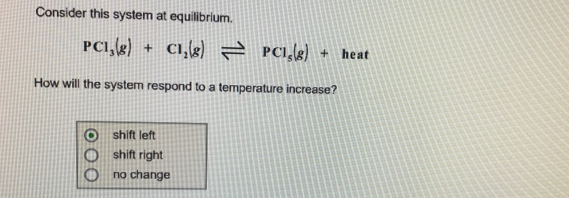Chemistry Practice Problems Le Chatelier's Principle Practice Problems Solution: Consider this system at equilibrium. PCl3(g) + Cl2...

# Solution: Consider this system at equilibrium. PCl3(g) + Cl2(g) ⇌ PCI5(g) + heat How will the system respond to a temperature increase? a. shift leftb. shift rightc. no change

###### Problem

Consider this system at equilibrium.

PCl3(g) + Cl2(g) ⇌ PCI5(g) + heat

How will the system respond to a temperature increase?

a. shift left

b. shift right

c. no changeView Complete Written Solution

Le Chatelier's Principle

Le Chatelier's Principle

#### Q. As the temperature of an endothermic equilibrium reaction increases, the equilibrium will shift to the left.a. Trueb. False

Solved • Tue Jul 24 2018 10:18:50 GMT-0400 (EDT)

Le Chatelier's Principle

#### Q.  Consider the following system at equilibrium.CaCl2 (s) ⇌ Ca2+ (aq) + 2Cl- (aq)1) How will adding more CaCl2 shift the equilibrium?      a) to the rig...

Solved • Tue Jul 03 2018 10:53:50 GMT-0400 (EDT)

Le Chatelier's Principle

#### Q. 2AB (aq) ⇌ A 2 (aq) B2 (aq)  The above reaction is at equilibrium. I begin to remove A 2 from the system. How does this affect equilibrium?

Solved • Mon Jun 25 2018 17:20:36 GMT-0400 (EDT)

Le Chatelier's Principle

#### Q. At a certain temperature, the equilibrium constant, K c, for the reaction Cl2(g) ⇌ 2Cl(g) is 5.92 x 104. A. If 2.77 g of Cl 2 are placed in a 2.50 L f...

Solved • Tue Jun 12 2018 13:40:25 GMT-0400 (EDT)